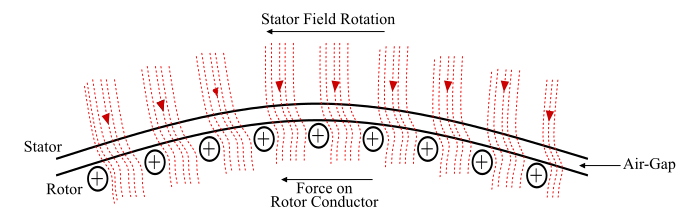# Three Phase Induction Motor â€“ Working Principle

A three phase induction motor has a stator and a rotor. The stator carries a 3-phase winding called as stator winding while the rotor carries a short circuited winding called as rotor winding. The stator winding is fed from 3-phase supply and the rotor winding derives its voltage and power from the stator winding through electromagnetic induction. Therefore, the working principle of a 3-phase induction motor is fundamentally based on electromagnetic induction.

Consider a portion of a three phase induction motor (see the figure). Therefore, the working of a three phase induction motor can be explained as follows −• When the stator winding is connected to a balanced three phase supply, a rotating magnetic field (RMF) is setup which rotates around the stator at synchronous speed (Ns). Where,

$$\mathrm{N_{S}=\frac{120f}{P}}$$

• The RMF passes through air gap and cuts the rotor conductors, which are stationary at start. Due to relative motion between RMF and the stationary rotor, an EMF is induced in the rotor conductors. Since the rotor circuit is short-circuited, a current starts flowing in the rotor conductors.

• Now, the current carrying rotor conductors are in a magnetic field created by the stator. As a result of this, mechanical force acts on the rotor conductors. The sum of mechanical forces on all the rotor conductors produces a torque which tries to move the rotor in the same direction as the RMF.

• Hence, the induction motor starts to rotate. From, the above discussion, it can be seen that the three phase induction motor is self-starting motor.

• The three induction motor accelerates till the speed reached to a speed just below the synchronous speed.